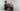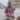# 7 Interview Questions on JavaScript Closures. Can You Answer Them?

Posted February 9, 2021Start discussion

Every JavaScript developer must know what a closure is. During a JavaScript coding interview there’s a good chance you’ll get asked about the concept of closures.

I compiled a list of 7 interesting and increasingly challenging questions on JavaScript closures.

Take a pencil and a piece of paper, and try to answer the questions without looking at the answers, or running the code. In my estimation, you would need about 30 minutes.

Have fun!

If you need a refresh on closures, I recommend checking the post A Simple Explanation of JavaScript Closures.

## Questions 1: Closures raise your hand

Consider the following functions `clickHandler`, `immediate`, and `delayedReload`:

``````let countClicks = 0;
countClicks++;
});``````
``````const result = (function immediate(number) {
const message = `number is: \${number}`;
return message;
})(100);``````
``````setTimeout(function delayedReload() {
}, 1000);``````

Which of these 3 functions access outer scope variables?

1. `clickHandler` accesses the variable `countClicks` from the outer scope.
2. `immediate` doesn’t access any variables from the outer scope.
3. `delayedReload` accesses the global variable `location` from the global scope (aka the outermost scope).

## Questions 2: Lost in parameters

What will log to console the following code snippet:

``````(function immediateA(a) {
return (function immediateB(b) {
console.log(a); // What is logged?
})(1);
})(0);``````

`0` is logged to the console. Try the demo.

`immediateA` is called with the argument `0`, thus `a` parameter is `0`.

`immediateB` function, being nested into `immediateA` function, is a closure that captures `a` variable from the outer `immediateA` scope, where `a` is `0`. Thus `console.log(a)` logs `0`.

## Questions 3: Who’s who

What will log to console the following code snippet:

``````let count = 0;
(function immediate() {
if (count === 0) {
let count = 1;
console.log(count); // What is logged?
}
console.log(count); // What is logged?
})();``````

`1` and `0` is logged to the console. Try the demo.

The first statement `let count = 0` declares a variable `count`.

`immediate()` is a closure that captures the `count` variable from the outer scope. Inside of the `immediate()` function scope `count` is `0`.

However, inside the conditional, another `let count = 1` declares a local variable `count`, which overwrites `count` from outer the scope. The first `console.log(count)` logs `1`.

The second `console.log(count)` logs `0`, since here `count` variable is accessed from the outer scope.

## Questions 4: Tricky closure

What will log to console the following code snippet:

``````for (var i = 0; i < 3; i++) {
setTimeout(function log() {
console.log(i); // What is logged?
}, 1000);
}``````

`3`, `3`, `3` is logged to console. Try the demo.

The code snippet executes in 2 phases.

Phase 1

1. `for()` iterating 3 times. During each iteration a new function `log()` is created, which captures the variable `i`. `setTimout()` schedules `log()` for execution after 1000ms.
2. When `for()` cycle completes, `i` variable has value `3`.

Phase 2

The second phase happens after 1000ms:

1. `setTimeout()` executes the scheduled `log()` functions. `log()` reads the current value of variable `i`, which is `3`, and logs to console `3`.

That’s why `3`, `3`, `3` is logged to the console.

Side challenge: how would you fix this example to log `0`, `1`, `2` values? Write your solution in a comment below!

## Questions 5: Right or wrong message

What will log to console the following code snippet:

``````function createIncrement() {
let count = 0;
function increment() {
count++;
}

let message = `Count is \${count}`;
function log() {
console.log(message);
}

return [increment, log];
}

const [increment, log] = createIncrement();
increment();
increment();
increment();
log(); // What is logged?``````

`'Count is 0'` is logged to console. Try the demo.

`increment()` function has been called 3 times, effectively incrementing `count` to value `3`.

`message` variable exists within the scope of `createIncrement()` function. Its initial value is `'Count is 0'`. However, even if `count` variable has been incremented a few times, `message` variable always holds `'Count is 0'`.

`log()` function is a closure that captures `message` variable from the `createIncrement()` scope. `console.log(message)` logs `'Count is 0'` to console.

Side challenge: how would you fix `log()` function to return the message having the actual `count` value? Write your solution in a comment below!

## Questions 6: Restore encapsulation

The following function `createStack()` creates instances of stack data structure:

``````function createStack() {
return {
items: [],
push(item) {
this.items.push(item);
},
pop() {
return this.items.pop();
}
};
}

const stack = createStack();
stack.push(10);
stack.push(5);
stack.pop(); // => 5

stack.items; // => 
stack.items = [10, 100, 1000]; // Encapsulation broken!``````

The stack works as expected, but with one small problem. Anyone can modify items array directly because `stack.items` property is exposed.

That’s an issue since it breaks the encapsulation of the stack: only `push()` and `pop()` methods should be public, but `stack.items` or any other details shouldn’t be accessible.

Refactor the above stack implementation, using the concept of closure, such that there is no way to access `items` array outside of `createStack()` function scope:

``````function createStack() {
}

const stack = createStack();
stack.push(10);
stack.push(5);
stack.pop(); // => 5

stack.items; // => undefined``````

Here’s a possible refactoring of `createStack()`:

``````function createStack() {
const items = [];
return {
push(item) {
items.push(item);
},
pop() {
return items.pop();
}
};
}

const stack = createStack();
stack.push(10);
stack.push(5);
stack.pop(); // => 5

stack.items; // => undefined``````

Try the demo.

`items` has been moved to a variable inside `createStack()` scope.

Thanks to this change, from the outside of `createStack()` scope, there is no way to access or modify `items` array. `items` is now a private variable, and the stack is encapsulated: only `push()` and `pop()` method are public.

`push()` and `pop()` methods, being closures, capture `items` variable from `createStack()` function scope.

## Questions 7: Smart multiplication

Write a function `multiply()` that multiples 2 numbers:

``````function multiply(num1, num2) {
}``````

If `multiply(num1, numb2)` is invoked with 2 arguments, it should return the multiplication of the 2 arguments.

But if invoked with 1 argument `const anotherFunc = multiply(num1)`, the function should return another function. The returned function when called `anotherFunc(num2)` performs the multiplication `num1 * num2`.

``````multiply(4, 5); // => 20
multiply(3, 3); // => 9

const double = multiply(2);
double(5);  // => 10
double(11); // => 22``````

Here’s a possible implementation of `multiply()` function:

``````function multiply(number1, number2) {
if (number2 !== undefined) {
return number1 * number2;
}
return function doMultiply(number2) {
return number1 * number2;
};
}

multiply(4, 5); // => 20
multiply(3, 3); // => 9

const double = multiply(2);
double(5);  // => 10
double(11); // => 22``````

Try the demo.

If `number2` parameter is not `undefined`, then the function simply returns `number1 * number2`.

But if `number2` is `undefined`, it means that `multiply()` function has been called with one argument. In such a case let’s return a function `doMultiply()` that when later invoked performs the actual multiplication.

`doMultiply()` is a closure because it captures `number1` variable from `multiply()` scope.

## Summary

• You have a good understanding of closures if you answered correctly 5 or more questions
• But you need a good refresher on closures if you answered correctly less than 5 questions. I recommend checking my post A Simple Explanation of JavaScript Closures.

Ready for a new challenge? Try to solve the 7 Interview Questions on “this” keyword in JavaScript.

## Quality posts into your inbox

I regularly publish posts containing:

• Important JavaScript concepts explained in simple words
• Overview of new JavaScript features
• How to use TypeScript and typing
• Software design and good coding practices